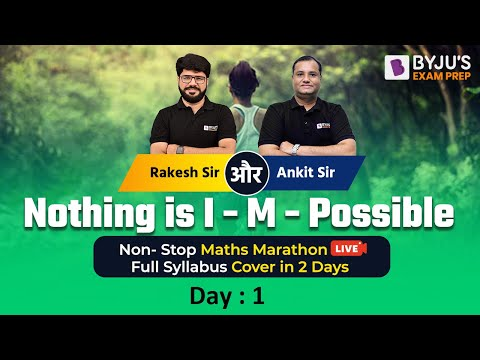# Engineering Mathematics for GATE

Engineering Mathematics is an important section in the GATE Exam as it carries around 15 marks out of 100. If the aspirants manage to prepare well, it will be easy for them to score well in GATE 2023.

Get all the necessary information like syllabus, exam pattern, new changes introduced in GATE, important books, preparation tips, previous years’ question papers, and the latest news related to the GATE Engineering Mathematics section here.

## GATE Engineering Mathematics Latest News

GATE 2023 exams will be held on 4, 5, 11, and 12 February 2023 are now available to access the GATE Answer Key and GATE Syllabus from their GOAPS Portal.

### GATE Engineering Mathematics Overview

 Particulars Details Number of Questions in Paper 65 Number of Sections Section 1 – General Aptitude, Section 2 – Engineering Mathematics and Subject of the Paper Topic-wise Weightage General Aptitude – 15% Engineering Mathematics – 15% Subject of the paper – 70% Pattern of Questions Multiple Choice Question (MCQs) and Numerical Answer Type Questions (NATQs) Duration of Exam 3 Hours Marking Scheme Test Paper has questions carrying 1 mark and 2 marks, and ⅓ and ⅔ negative mark for incorrect answers, respectively

## GATE Engineering Mathematics Preparation Videos

Preparation and Expected Questions:Complete Revision:## GATE Engineering Mathematics Important Dates 2023

 Particulars Dates GATE Online Application Processing 30th August, 2022 Closing of submission of Application Form 30th September, 2022 Last Date of Extended period for Online Registration Application (With Late Fees) 7th October, 2022 Last date for requesting a change of examination city 4th to 11th November, 2022 Admit Card 3rd January 2023 GATE 2023 Examination 4th, 5th, 11th and 12th February, 2023 Announcement of Result 16th March, 2023

## GATE Engineering Mathematics Exam Pattern

Candidates can check the overview of the GATE Exam Pattern for EE in the table below:

 Feature Description Examination Mode Online (Computer Based Test) Duration of Exam 3 Hours (180 Minutes) Sectional Time Duration None Total Marks 100 Total Number of Questions 65 Number of Subjects (Papers) 29 Number of Sections It depends on the subjects. 2-3 (GA and Core Discipline or GA, Engineering Mathematics and Core Subject) Type of Questions Multiple Choice Questions (MCQ) Multiple Select Questions (MSQ) Numerical Answer Type (NAT) Questions Marking Scheme 1 or 2 marks for each right answer Negative Marking Scheme For a wrong answer chosen in a MCQ, there will be negative marking. For 1-mark MCQ, 1/3 mark will be deducted for a wrong answer. For 2-mark MCQ, 2/3 mark will be deducted for a wrong answer. No negative marking for MSQ & NATQs.

Marking Scheme for Engineering Mathematics:

 Paper Exam Pattern Mechanical Engineering (ME) GA (General Aptitude) – 15 marks Engineering Mathematics – 13 marks Subject of the Paper – 72 marks

## GATE Engineering Mathematics Important Topics for Exam Preparation

 SN Topic Particulars Details 1. Linear Algebra (for all GATE streams) Important Topics Eigenvalues and Vectors Finding rank and determinant of matrices Systems of Linear Equations Model Questions Find the Matrix for the given Eigenvalues and Eigenvectors Properties of Eigenvalues for Symmetric Matrices Find the rank and determinant of the given Matrix Properties of Matrices based on Determinants, Rank, etc. Find the solution for the given system of Linear Equations 2. Calculus (for all streams, some of the topics mentioned in the table are not required in all streams) Important Topic Limit Maxima and Minima Gradient, Divergence, and Curl (Vector Analysis in GATE EC) Model Questions Find the maximum and minimum values for the given functions Simple questions on limit and continuity Find the Divergence of the given Vector field 3. Differential Calculus (for all GATE streams) Important Topic First-order equations (Linear and Nonlinear) Cauchy’s and Euler’s equations Model Questions Find the solution of given Differential Equation 4. Complex Analysis (except GATE CS) Important Topic Analytic Functions Cauchy-Riemann Equations Taylor’s Series Model Questions Find the expression for one of u(x,y) and v(x,y), for the given analytical function f(z) = u(x,y) + iv(x, y) and also provided value of i with other expression Integration of given complex function in either counterclockwise or anticlockwise direction 5. Numerical Methods (except GATE CS) Important Topic Newton-Raphson Method Integration by trapezoidal and Simpso’s rules Model Questions Find the iteration value of the equation using Newton-Raphson method Find the value of the given integral using Trapezoidal and Simpson’s rules 6. Probability and Statistics (for all Gate streams) Important Topic Joint and Conditional Probability Uniform, Normal, Exponential Distributions Model Questions Finding the probability for coin-based problems, dice-based problems, etc. Finding the probability using distributions 7. Transform Theory (for GATE EE and ME) Important Topic Laplace Transformation Model Question Find the Laplace Transform and Inverse Laplace transform of the given function 8. Discrete Mathematics (only for GATE CS) Important Topic Mathematical Logic (Propositions and Predicate logic) Relations and Functions Graph Connectivity and Colouring Model Question Find the first-order logic sentence for the given English statement and with predicates Finding tautology, satisfiability, equivalences of given propositional statements Find the given relation is reflexive, symmetric, transitive, equivalence, etc. Finding the number of edges, vertices, or components for the given connected or disconnected graph Checking isomorphism, Euler circuit, Hamiltonian cycle for given graphs Simple properties of various graphs, such as a complete graph, bipartite graph, regular graph, cycle graph and line graph

Also, explore the pages below for GATE Engineering Mathematics preparation:

Q1

### Is Engineering Maths easy in GATE?

For GATE 2022, it was quite challenging.

Q2

### Is Engineering Maths the same for all branches in GATE?

It is the same for subject codes, CE, CS, EC, AE, AG, BT, CH, ME, MN, MT, EE, IN, TF, XE, PE, ST, and PI.

Q3

### What is the weightage of Engineering Mathematics in GATE?

It is 15-13% for subject codes, CE, CS, EC, AE, AG, BT, CH, ME, MN, MT, EE, IN, TF, XE, PE, ST, and PI.